# 聚类分析

### K 均值和层次聚类

Statistics and Machine Learning Toolbox 中的一些函数可执行 K 均值聚类和层次聚类。

K 均值聚类是一种分区方法，它将数据中的观测值视为具有位置和相互间距离的对象。它将对象划分为 K 个互斥簇，使每个簇中的对象尽可能彼此靠近，并尽可能远离其他簇中的对象。每个簇的特性由其质心或中心点决定。当然，聚类中使用的距离通常不代表空间距离。

```rng(6,'twister') ```

### Fisher 鸢尾花数据

20 世纪 20 年代，植物学家收集了 150 个鸢尾花标本（三个品种各取 50 个标本）的萼片长度、萼片宽度、花瓣长度和花瓣宽度的测量值。这些测量值被称为 Fisher 鸢尾花数据集。

### 使用 K 均值聚类方法对 Fisher 鸢尾花数据进行聚类

```load fisheriris [cidx2,cmeans2] = kmeans(meas,2,'dist','sqeuclidean'); [silh2,h] = silhouette(meas,cidx2,'sqeuclidean'); ```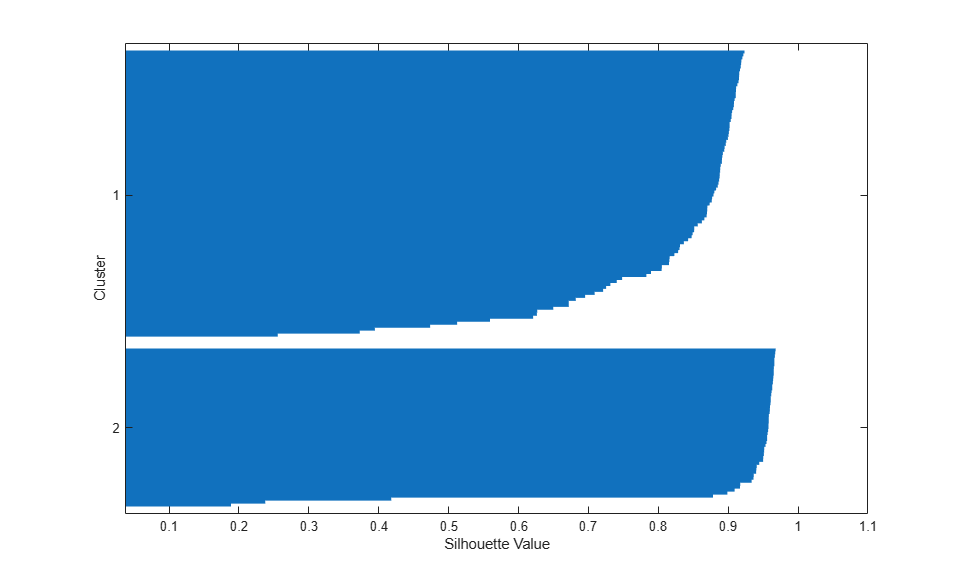```ptsymb = {'bs','r^','md','go','c+'}; for i = 1:2 clust = find(cidx2==i); plot3(meas(clust,1),meas(clust,2),meas(clust,3),ptsymb{i}); hold on end plot3(cmeans2(:,1),cmeans2(:,2),cmeans2(:,3),'ko'); plot3(cmeans2(:,1),cmeans2(:,2),cmeans2(:,3),'kx'); hold off xlabel('Sepal Length'); ylabel('Sepal Width'); zlabel('Petal Length'); view(-137,10); grid on ```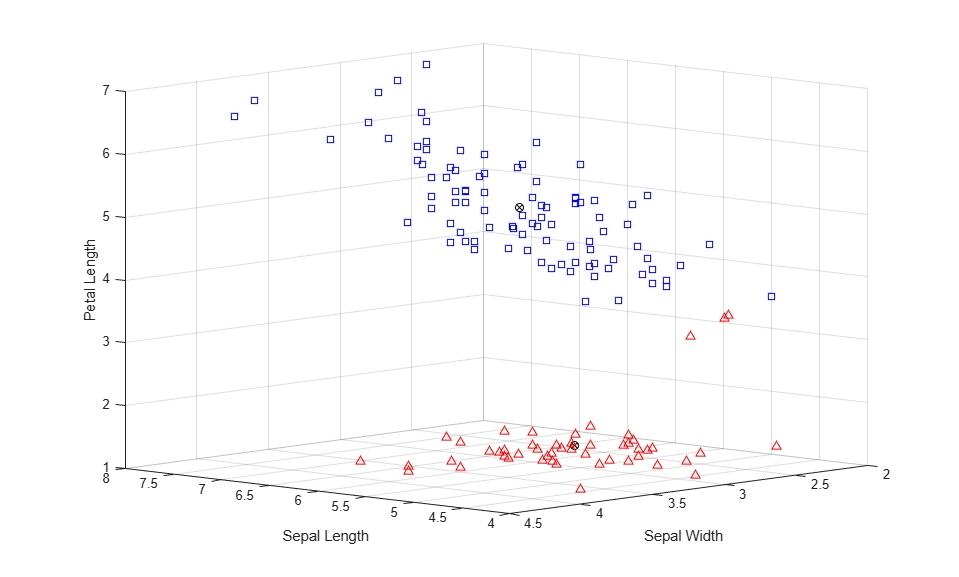```[cidx3,cmeans3] = kmeans(meas,3,'Display','iter'); ```
``` iter phase num sum 1 1 150 146.424 2 1 5 144.333 3 1 4 143.924 4 1 3 143.61 5 1 1 143.542 6 1 2 143.414 7 1 2 143.023 8 1 2 142.823 9 1 1 142.786 10 1 1 142.754 Best total sum of distances = 142.754 ```

```[cidx3,cmeans3,sumd3] = kmeans(meas,3,'replicates',5,'display','final'); ```
```Replicate 1, 9 iterations, total sum of distances = 78.8557. Replicate 2, 10 iterations, total sum of distances = 78.8557. Replicate 3, 8 iterations, total sum of distances = 78.8557. Replicate 4, 8 iterations, total sum of distances = 78.8557. Replicate 5, 1 iterations, total sum of distances = 78.8514. Best total sum of distances = 78.8514 ```

```sum(sumd3) ```
```ans = 78.8514 ```

```[silh3,h] = silhouette(meas,cidx3,'sqeuclidean'); ```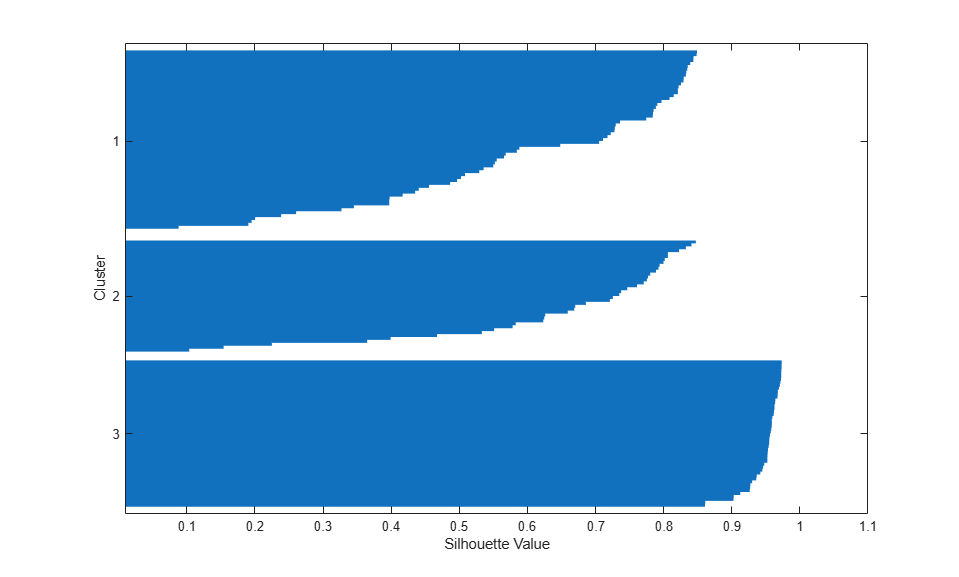```for i = 1:3 clust = find(cidx3==i); plot3(meas(clust,1),meas(clust,2),meas(clust,3),ptsymb{i}); hold on end plot3(cmeans3(:,1),cmeans3(:,2),cmeans3(:,3),'ko'); plot3(cmeans3(:,1),cmeans3(:,2),cmeans3(:,3),'kx'); hold off xlabel('Sepal Length'); ylabel('Sepal Width'); zlabel('Petal Length'); view(-137,10); grid on ```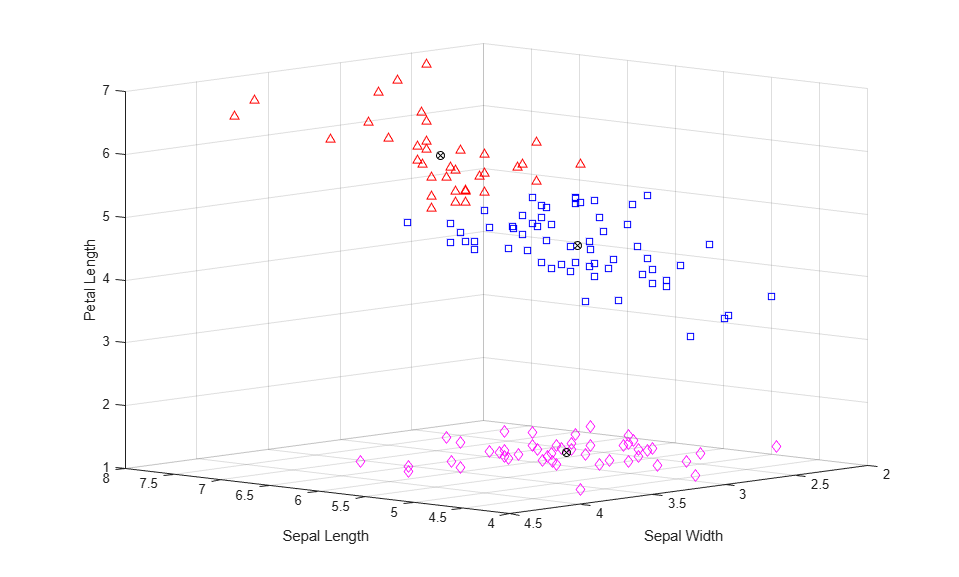```[mean(silh2) mean(silh3)] ```
```ans = 0.8504 0.7357 ```

```[cidxCos,cmeansCos] = kmeans(meas,3,'dist','cos'); ```

```[silhCos,h] = silhouette(meas,cidxCos,'cos'); [mean(silh2) mean(silh3) mean(silhCos)] ```
```ans = 0.8504 0.7357 0.7491 ```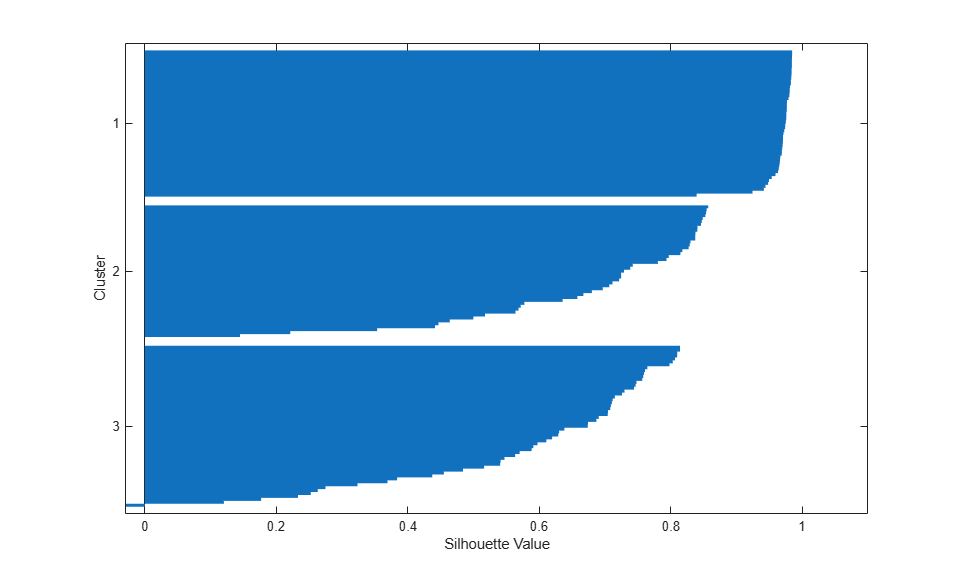```for i = 1:3 clust = find(cidxCos==i); plot3(meas(clust,1),meas(clust,2),meas(clust,3),ptsymb{i}); hold on end hold off xlabel('Sepal Length'); ylabel('Sepal Width'); zlabel('Petal Length'); view(-137,10); grid on ```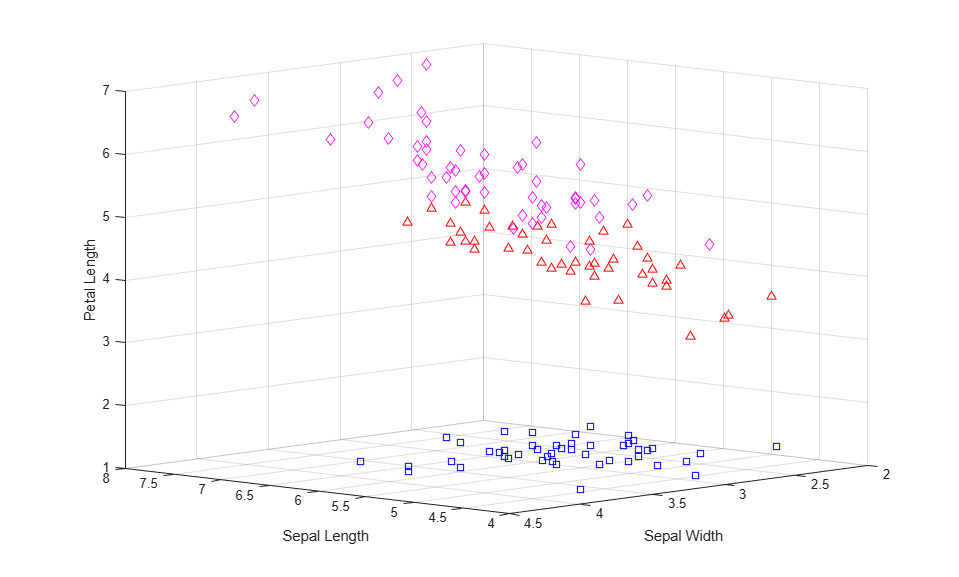```lnsymb = {'b-','r-','m-'}; names = {'SL','SW','PL','PW'}; meas0 = meas ./ repmat(sqrt(sum(meas.^2,2)),1,4); ymin = min(min(meas0)); ymax = max(max(meas0)); for i = 1:3 subplot(1,3,i); plot(meas0(cidxCos==i,:)',lnsymb{i}); hold on; plot(cmeansCos(i,:)','k-','LineWidth',2); hold off; title(sprintf('Cluster %d',i)); xlim([.9, 4.1]); ylim([ymin, ymax]); h_gca = gca; h_gca.XTick = 1:4; h_gca.XTickLabel = names; end ```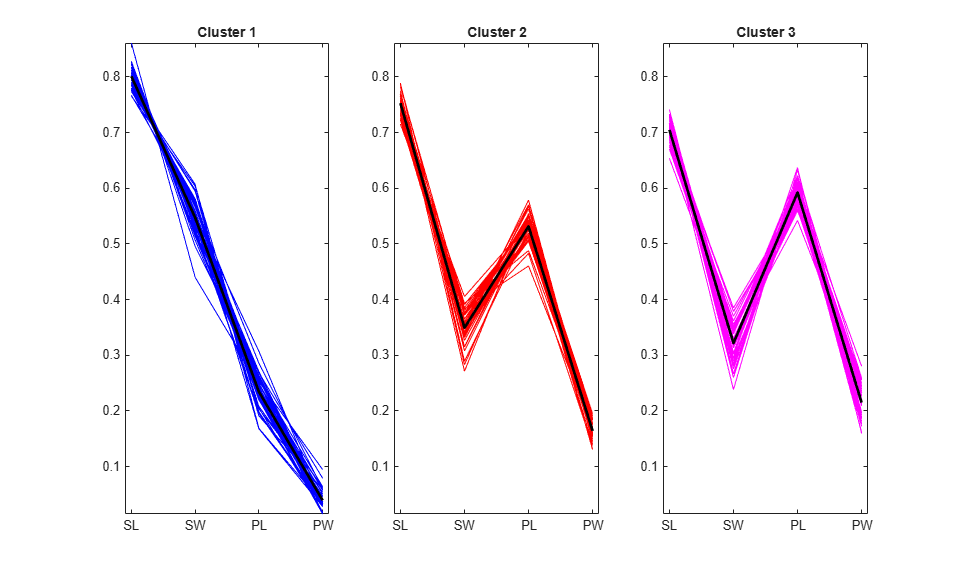```subplot(1,1,1); for i = 1:3 clust = find(cidxCos==i); plot3(meas(clust,1),meas(clust,2),meas(clust,3),ptsymb{i}); hold on end xlabel('Sepal Length'); ylabel('Sepal Width'); zlabel('Petal Length'); view(-137,10); grid on sidx = grp2idx(species); miss = find(cidxCos ~= sidx); plot3(meas(miss,1),meas(miss,2),meas(miss,3),'k*'); legend({'setosa','versicolor','virginica'}); hold off ```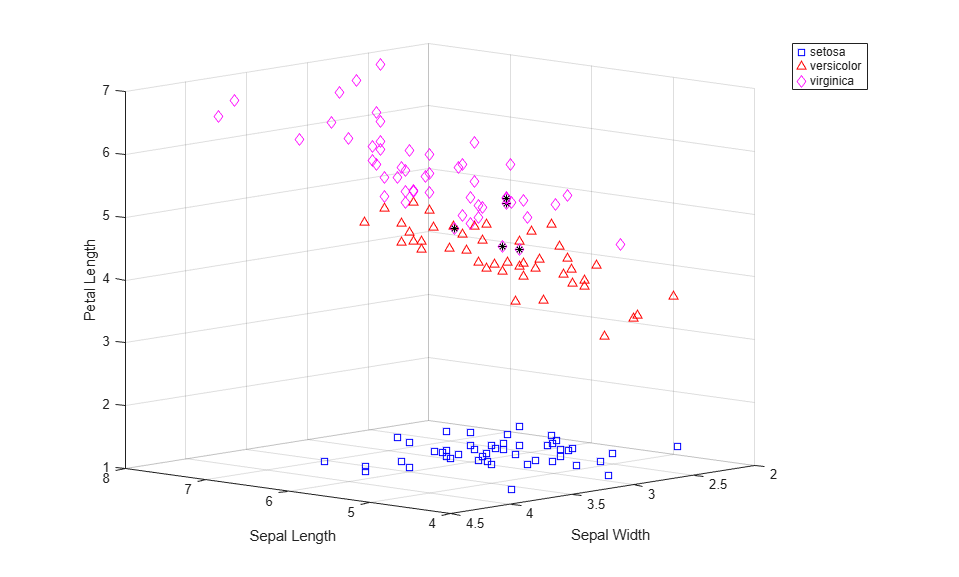### 使用层次聚类方法对 Fisher 鸢尾花数据进行聚类

K 均值聚类只产生一个鸢尾花数据分区，但您可能还想按照不同的分组尺度来研究数据。要实现这一点，您可以通过层次聚类创建层次聚类树。

```eucD = pdist(meas,'euclidean'); clustTreeEuc = linkage(eucD,'average'); ```

```cophenet(clustTreeEuc,eucD) ```
```ans = 0.8770 ```

```[h,nodes] = dendrogram(clustTreeEuc,0); h_gca = gca; h_gca.TickDir = 'out'; h_gca.TickLength = [.002 0]; h_gca.XTickLabel = []; ```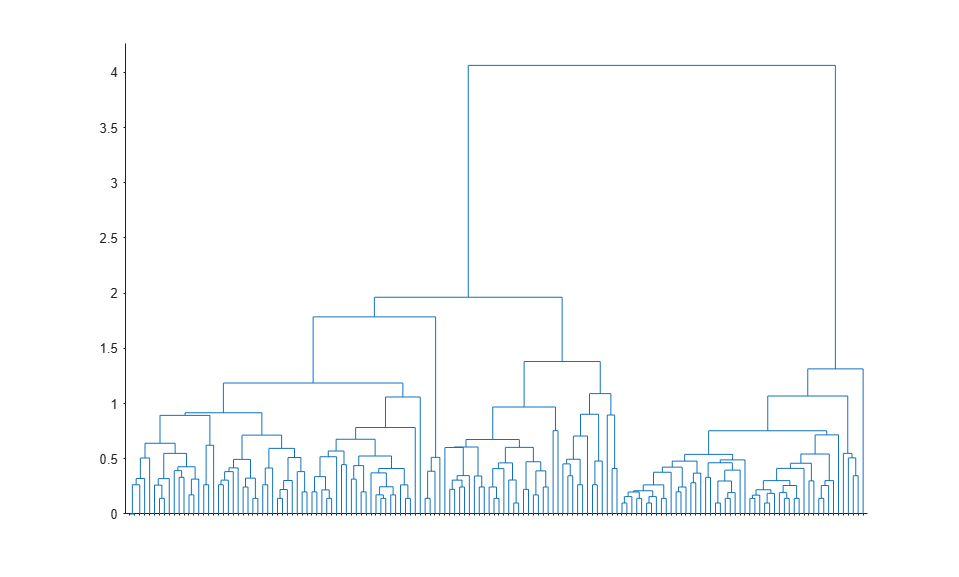```cosD = pdist(meas,'cosine'); clustTreeCos = linkage(cosD,'average'); cophenet(clustTreeCos,cosD) ```
```ans = 0.9360 ```
```[h,nodes] = dendrogram(clustTreeCos,0); h_gca = gca; h_gca.TickDir = 'out'; h_gca.TickLength = [.002 0]; h_gca.XTickLabel = []; ```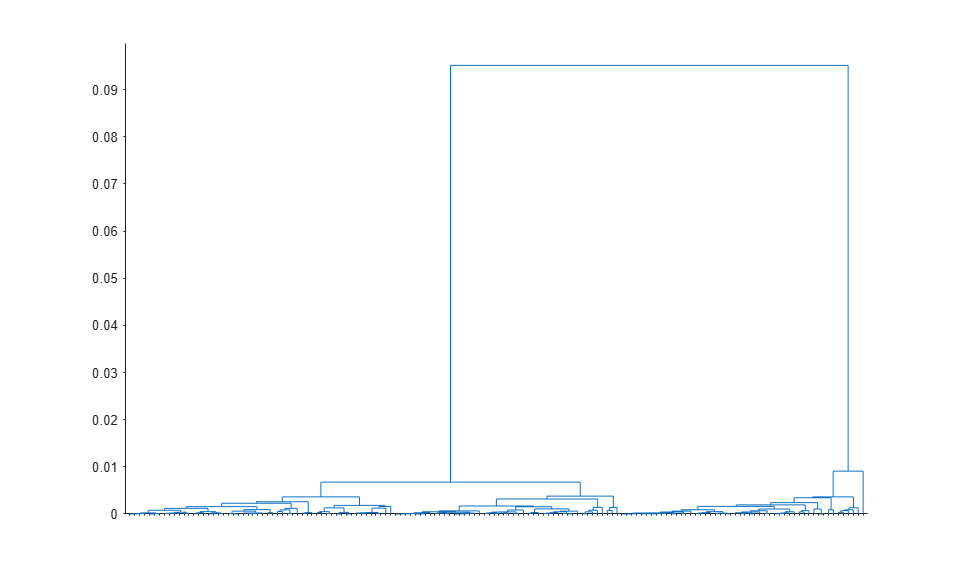```[h,nodes] = dendrogram(clustTreeCos,12); ```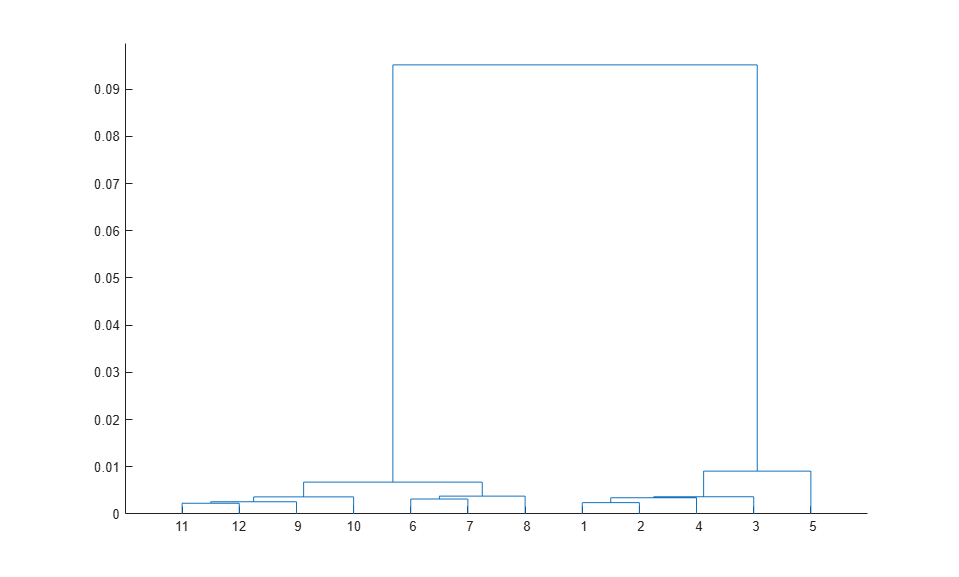```[sum(ismember(nodes,[11 12 9 10])) sum(ismember(nodes,[6 7 8])) ... sum(ismember(nodes,[1 2 4 3])) sum(nodes==5)] ```
```ans = 54 46 49 1 ```

```hidx = cluster(clustTreeCos,'criterion','distance','cutoff',.006); for i = 1:5 clust = find(hidx==i); plot3(meas(clust,1),meas(clust,2),meas(clust,3),ptsymb{i}); hold on end hold off xlabel('Sepal Length'); ylabel('Sepal Width'); zlabel('Petal Length'); view(-137,10); grid on ```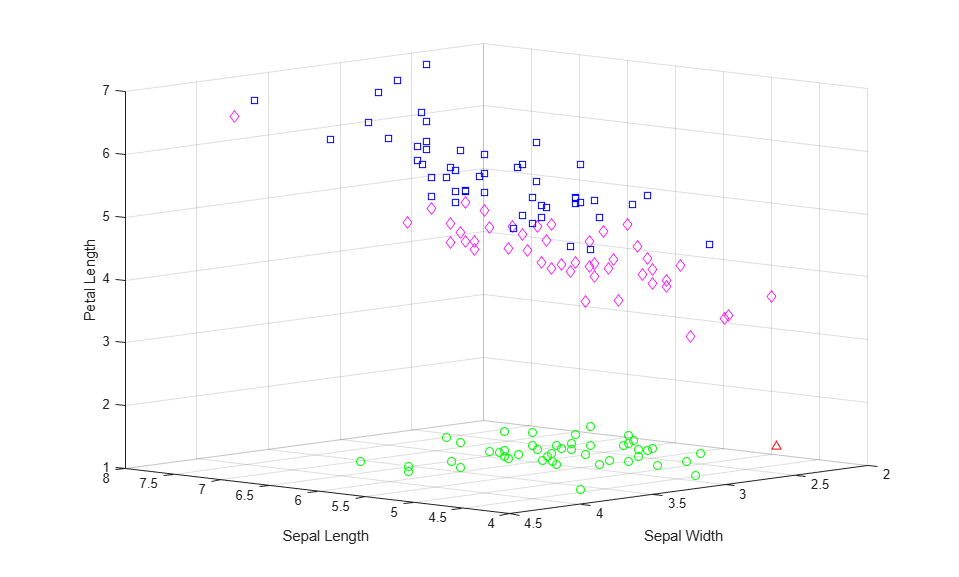```clustTreeSng = linkage(eucD,'single'); [h,nodes] = dendrogram(clustTreeSng,0); h_gca = gca; h_gca.TickDir = 'out'; h_gca.TickLength = [.002 0]; h_gca.XTickLabel = []; ```